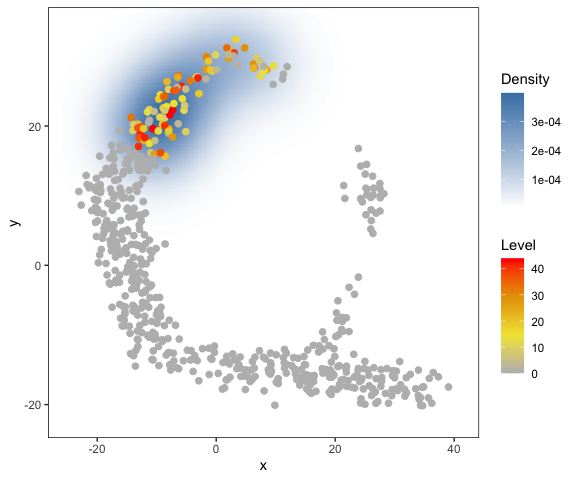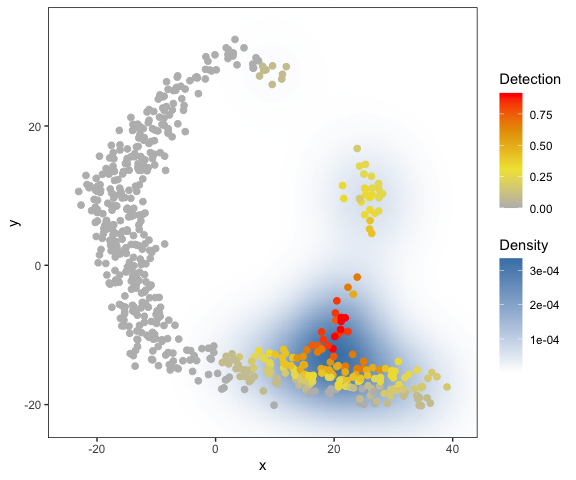# Application on a toy dataset

A small toy dataset is included in the package. The toy dataset includes:

• dat.expression: a toy scRNA-seq dataset with genes (rows) and cells (columns)

• dat.tsne: 2D coordinates of the cells in a t-SNE splot

First, let’s apply haystack (the main function of the package) on the toy dataset. This should take just several seconds on a typical desktop computer.

library(singleCellHaystack)
set.seed(1234)

# Turn the expression data into detection (gene detected = TRUE, not detected = FALSE)
# let's define detection as having more than 1 read
dat.detection <- dat.expression > 1

# run the main 'haystack' analysis
# inputs are:
# 1) the coordinates of the cells in the input space (here: dat.tsne)
# 2) the detection data (dat.detection)
# 3) the method used ("2D" since we are using a 2D input space here)
res <- haystack(dat.tsne, detection = dat.detection, method = "2D")
#> ### calling haystack_2D()...
#> ### setting parameters...
#> ### calculating Kullback-Leibler divergences...
#> ### performing randomizations...
#> ### estimating p-values...
#> ### returning result...

# the returned results 'res' is of class 'haystack'
class(res)
#>  "haystack"

Let’s have a look at the most significant differentially expressed genes (DEGs).

# show top 10 DEGs
show_result_haystack(res.haystack = res, n=10)
#>              D_KL log.p.vals log.p.adj T.counts
#> gene_497 1.599646  -25.55327 -22.85430       86
#> gene_79  1.929800  -24.93934 -22.24037       68
#> gene_62  1.519993  -24.68869 -21.98972       81
#> gene_61  1.470475  -24.12825 -21.42928       80
#> gene_339 1.414814  -23.87108 -21.17211       87
#> gene_351 1.408946  -23.62384 -20.92487       89
#> gene_137 1.349994  -23.08019 -20.38122       89
#> gene_444 1.435882  -22.92343 -20.22446       75
#> gene_317 1.386194  -22.38427 -19.68530       94
#> gene_313 1.294544  -22.16986 -19.47089       78

# alternatively: use a p-value threshold
#show_result_haystack(res.haystack = res, p.value.threshold = 1e-10)

One of the most significant DEGs is “gene_497”. Here we visualize its expression in the t-SNE plot. As you can see, this DEG is expressed only in cells in the upper-left corner of the plot.

# visualize one of the surprizing genes
plot_gene_haystack(
dat.tsne,
expression = dat.expression,
gene = "gene_497",
detection = dat.detection,
high.resolution = TRUE,
point.size = 2
)Yes, the coordinates of the cells in this toy example t-SNE space roughly resemble a haystack; see the Haystack paintings by Monet.

# Clustering and visualization

You are not limited to single genes. Here, we pick up a set of DEGs, and group them by their expression pattern in the plot into 5 clusters.

# get the top most significant genes, and cluster them by their distribution pattern in the 2D plot
sorted.table <- show_result_haystack(res.haystack = res, p.value.threshold = 1e-10)
gene.subset <- row.names(sorted.table)

# k-means clustering
km <- kmeans_haystack(dat.tsne, detection=dat.detection, genes=gene.subset, k=5)
km.clusters <- km\$cluster

# alternatively: hierarchical clustering
#hc <- hclust_haystack(dat.tsne, detection=dat.detection, genes=gene.subset)
#hc.clusters <- cutree(hc,k = 5)

… and visualize the average pattern of the genes in cluster 1 (for example).

# visualize cluster distributions
plot_gene_set_haystack(dat.tsne, detection = dat.detection,
genes=names(km.clusters[km.clusters==1]), point.size=2)From this plot we can see that genes in cluster 1 are mainly expressed in cells in the lower right part of the plot.